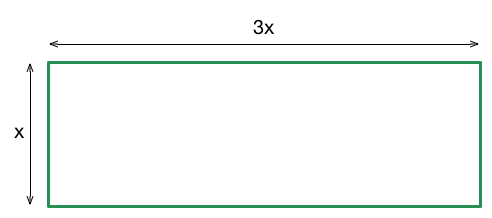# Perimeter from Area

Geometry Level 1The length of a rectangle is three times of its width. If the area of the rectangle is $300 \text{ m}^2$, what is the perimeter of the rectangle (in $\text{m}$)?

×